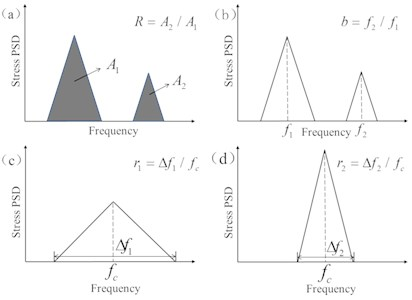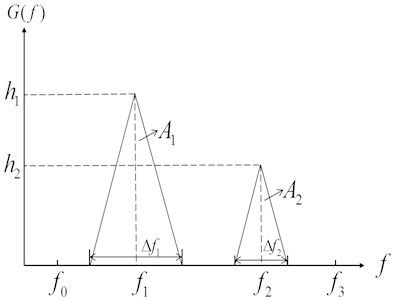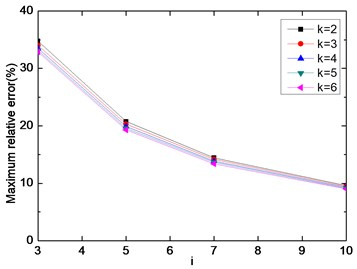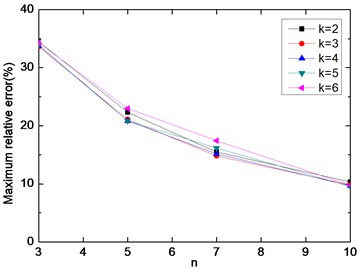Published: 07 May 2020

# A validation of the stress power spectral density method with zero-order moment

N. N. Zhuang1
H. B. Chen2
Y. Y. Wang3
1, 2, 3Department of Modern mechanics, University of Science and Technology of China, CAS Key Laboratory of Mechanical Behavior and Design of Materials, Hefei, China
3Chengdu Aircraft Design and Research Institute, Chengdu, China
Corresponding Author:
H. B. Chen
Views 181

#### Abstract

To give a fatigue life prediction for problems with bimodal stress power spectral density which is commonly observed in the dynamic response of structures, the bimodal triangular model is established first and a large sum of bimodal stress power spectral densities with different characteristics are built. Then the error analysis of the fatigue life prediction results of bimodal stress power spectral densities with different shapes are calculated by the stress power spectral density method with zero-order moment (SPSD0) and the classical frequency-domain fatigue life prediction method, which is under single frequency band process and broadband process. The simulation results show that, for single frequency band process or broadband process being divided into appropriate segments, the fatigue damage is not sensitive to the real curve shape of bimodal stress power spectral density.

## 1. Introduction

Fatigue damage to a structure has always been one of the most important damage factors in engineering [1-3]. According to statistics, 50 %-90 % of the damage of mechanical components is fatigue damage . During the service period of engineering structures, random loads are very common. Therefore, the fatigue life prediction and reliability prediction of the structure under random load is particularly important. The bimodal stress power spectral density is a very typical form of the structural dynamic response. It is characterized by two separate vibration modes , for example, the response of aircraft’s shell and plate subsystem under the excitation of complex pulsating noise. Its main shape of the stress power spectral density corresponds to the first two natural frequencies of the structure is shown as a double peak curve.

In recent years, through a large number of numerical simulations, Wang  found that fatigue analysis using the stress root mean square value of each frequency band usually has high engineering accuracy, based on the proposed SPSD0. However, he did not analyze the representative bimodal stress power spectral density. In this work, we selects the triangular model for the bimodal stress power spectral density. The fatigue life was predicted using the SPSD0, and compared with the fatigue life prediction results of Dirlik method. Thus, the validity of SPSD0 was further verified.

## 2. Fatigue life prediction method

### 2.1. Classical frequency-domain fatigue life prediction method (Dirlik method)

The frequency-domain fatigue life prediction method uses the structure’s stress power spectral density function to describe the stress amplitude or peak information in the frequency domain to obtain the probability density function. According to the S-N fatigue life curve of the material and the linear cumulative damage criterion, the fatigue life of a structure can be predicted. The frequency-domain fatigue life prediction method is simple in idea and has high calculation efficiency and is currently the most widely used fatigue life prediction method in engineering.

Dirlik  proposed a frequency domain fatigue life prediction method based on a large number of numerical simulation results, which is the most widely used in engineering. This method presents the probability density function of stress amplitude as a sum of an exponential distribution and two Rayleigh distributions :

1
${p}_{Dir}\left(S\right)=\frac{1}{\sqrt{{m}_{0}}}\left[\frac{{D}_{1}}{Q}{e}^{-\frac{Z}{Q}}+\frac{{D}_{2}Z}{{R}^{2}}{e}^{-\frac{{Z}^{2}}{2{R}^{2}}}+{D}_{3}Z{e}^{-\frac{{Z}^{2}}{2}}\right],$

where:

$Z=\frac{S}{\sqrt{{m}_{0}}},{D}_{1}=\frac{2\left({x}_{m}-{\alpha }_{2}^{2}\right)}{1+{\alpha }_{2}^{2}},{D}_{2}=\frac{1-{\alpha }_{2}^{2}-{D}_{1}+{D}_{1}^{2}}{1-R},{D}_{3}=1-{D}_{1}-{D}_{2},$
$Q=\frac{1.25\left({\alpha }_{2}-{D}_{3}-{D}_{2}R\right)}{{D}_{1}},R=\frac{{\alpha }_{2}-{x}_{m}-{D}_{1}^{2}}{1-{\alpha }_{2}-{D}_{1}+{D}_{1}^{2}},$
${x}_{m}={\alpha }_{1}\bullet {\alpha }_{2}=\frac{{m}_{1}}{\sqrt{{m}_{0}\bullet {m}_{2}}}\bullet \frac{{m}_{2}}{\sqrt{{m}_{0}\bullet {m}_{4}}}.$

The fatigue damage is:

2
${E\left[D\right]}_{Dir}=E\left[P\right]\frac{T}{C}{\int }_{0}^{\mathrm{\infty }}{S}^{k}{p}_{Dir}\left(S\right)dS,$

where $E\left[P\right]=\frac{1}{2\pi }\sqrt{\frac{{m}_{4}}{{m}_{2}}}$, ${m}_{i}$ is the $i$-th moment of the stress power spectral density function $G\left(f\right)$, ${m}_{i}=\int {f}^{i}G\left(f\right)df$.

### 2.2. The stress power spectral density method with zero-order moment (SPSD0) 

SPSD0 divides the total stress power spectral density into $N$ small frequency bands. The upper and lower frequency limits of the $i$-th small frequency band are ${f}_{Ui}$ (Upper frequency) and ${f}_{Li}$ (Lower frequency) respectively, and the $n$-th ($n=$ 0, 1, 2, 4) moment of the stress power spectral density of the $i$-th small frequency band is ${\left({m}_{n}\right)}_{i}$.

When the simplified stress power spectral density is used for calculation, the stress power spectral density in the small frequency band is a fixed value. If the value of the stress power spectral density in the $i$-th small frequency band is recorded as ${h}_{i}$, the following relationship is satisfied: ${\left({m}_{0}\right)}_{i}={\int }_{{f}_{Li}}^{{f}_{Ui}}{h}_{i}df={h}_{i}\left({f}_{Ui}-{f}_{Li}\right)$.

Therefore, ${h}_{i}=\frac{{\left({m}_{0}\right)}_{i}}{{f}_{Ui}-{f}_{Li}}$.

In turn, each moment of the entire stress power spectral density can be obtained.

Zero-order moment of stress power spectral density: ${m}_{0}=\sum _{i=1}^{N}{\left({m}_{0}\right)}_{i}$.

First moment of stress power spectral density:

${m}_{1}={\sum }_{i=1}^{N}{\int }_{{f}_{Li}}^{{f}_{Ui}}\left({h}_{i}\bullet f\right)df={\sum }_{i=1}^{N}\frac{{f}_{Ui}+{f}_{Li}}{2}{\left({m}_{0}\right)}_{i}.$

Second moment of stress power spectral density:

${m}_{2}={\sum }_{i=1}^{N}\frac{{f}_{Ui}^{2}+{f}_{Ui}{f}_{Li}+{f}_{Li}^{2}}{3}{\left({m}_{0}\right)}_{i}.$

Fourth moment of stress power spectral density:

${m}_{4}={\sum }_{i=1}^{N}\frac{{f}_{Ui}^{4}+{f}_{Ui}^{3}{{f}_{Li}+{f}_{Ui}^{2}{f}_{Li}^{2}+f}_{Ui}{f}_{Li}^{3}+{f}_{Li}^{4}}{5}{\left({m}_{0}\right)}_{i}.$

From the fatigue formula of the Dirlik method mentioned above, we know that the fatigue life can be predicted after zero, first, second and fourth order moments of the stress power spectral density are obtained. Here, SPSD0 combined with Dirlik method is called the zero-order moment Dirlik method, and the fatigue damage obtained is recorded as ${E\left[D\right]}_{Dir0}$.

Compared with the classical frequency-domain fatigue life prediction method, SPSD0 has its unique advantages: it is only necessary to know the zero-order moment of the stress power spectral density in each frequency band, in other words, without knowing the specific stress power spectral density the fatigue life prediction can be performed.

## 3. Bimodal stress power spectral density

The bimodal triangular stress power spectral density model (Fig. 1) can be formed by superimposing two triangular single peaks. On the whole, the overall shape depends on the specific characteristics of the two single peaks, specifically, the area of the two triangular spectra (${A}_{1}$or ${A}_{2}$), center frequency (${f}_{1}$ or ${f}_{2}$), area ratio $R$ (Fig. 1(a)), frequency ratio $b$ (Fig. 1(b)) and bandwidth ratio $r$ (Fig. 1(c)) and Fig. 1(d)): $R={A}_{2}/{A}_{1}$, $b={f}_{2}/{f}_{1}$, $r=∆f/{f}_{c}$.

Fig. 1The triangular model of bimodal stress power spectral densityFig. 2The bimodal stress power spectral densityIn the formula, ${A}_{1}$and ${A}_{2}$ are the areas of the first and second peaks, ${f}_{1}$ and ${f}_{2}$ are the center frequencies of the first and second peaks, respectively. By changing the base and height of the peak, single peaks with the same area but different shapes can be obtained.

In this paper, the calculation is mainly carried out by numerical simulation on the premise of ensuring that the zero-order moment of the stress power spectral density in the frequency band is a fixed value. The effect of the shape of bimodal stress power spectral density on the amount of fatigue damage is examined. For the stress power spectral density in the frequency band ${f}_{0}~{f}_{3}$, the shape of bimodal stress power spectral density is shown in Fig. 2, and the corresponding stress power spectral density function equation is as follows:

3
$G\left(f\right)=\left\{\begin{array}{ll}0,& {f}_{0}\le f\le {f}_{1}-{d}_{1},\\ \frac{{h}_{1}}{{d}_{1}}f+\frac{{{d}_{1}-f}_{1}}{{d}_{1}}{h}_{1},& {f}_{1}-{d}_{1}\le f\le {f}_{1},\\ -\frac{{h}_{1}}{{d}_{1}}f+\frac{{{d}_{1}+f}_{1}}{{d}_{1}}{h}_{1},& {f}_{1}\le f\le {f}_{1}+{d}_{1},\\ 0,& {f}_{1}+{d}_{1}\le f\le {f}_{2}-{d}_{2},\\ \frac{{h}_{2}}{{d}_{2}}f+\frac{{{d}_{2}-f}_{2}}{{d}_{2}}{h}_{2},& {f}_{2}-{d}_{2}\le f\le {f}_{2},\\ -\frac{{h}_{2}}{{d}_{2}}f+\frac{{{d}_{2}+f}_{2}}{{d}_{2}}{h}_{2},& {f}_{2}\le f\le {f}_{2}+{d}_{2},\\ 0,& {f}_{2}+{d}_{2}\le f\le {f}_{3},\end{array}\right\$

where:

${d}_{1}=\frac{∆{f}_{1}}{2}=\frac{{r}_{1}{f}_{1}}{2},{d}_{2}=\frac{∆{f}_{2}}{2}=\frac{{r}_{2}{f}_{2}}{2}=\frac{{r}_{2}{bf}_{1}}{2},$

and (${r}_{1}$and ${r}_{2}$ are the bandwidth ratios of the two peaks):

${h}_{1}=\frac{2{A}_{1}}{{r}_{1}{f}_{1}},{h}_{2}=\frac{2{A}_{2}}{{r}_{2}{f}_{2}}=\frac{2{RA}_{1}}{{r}_{2}{bf}_{1}}.$

## 4. Fatigue life prediction analysis

### 4.1. Sensitivity analysis of fatigue damage in single frequency band process

In the frequency band ${f}_{0}~{f}_{3}$, the zero-order moment of the stress power spectral density is a fixed value, that is ${A}_{1}+{A}_{2}=\left(1+R\right){A}_{1}={m}_{0}$, and ${A}_{1}=\frac{1}{1+R}{m}_{0}$ can be obtained. Combining with the equation of the double-peak stress power spectral density in Eq. (3), we can see that the specific shape of the stress power spectral density can be determined by the parameters ${f}_{1}$, $b$, $R$, ${r}_{1}$ and ${r}_{2}$.

Considering the influence of randomness to construct the bimodal stress power spectral density, when the parameters take different values, the specific stress power spectral density constructed also varies. The relative variables are chosen as the following rules: the frequency band ${f}_{0}~{f}_{3}$ is divided into $N$ equal parts with the same frequency resolution as $1/200{f}_{0}$, and the position of ${f}_{1}$ locates at each $N$ equal point. Once ${f}_{1}$ determined, divide the frequency band ${f}_{1}~{f}_{3}$ into six equal parts, and the position of ${f}_{2}$ locates at each six equal point, then, the frequency ratio $b$ picks 5 values in the interval $\left({1,f}_{3}/{f}_{1}\right)$. In order to ensure that each single peak is a narrow-band spectrum, the bandwidth ratios ${r}_{1}$ and ${r}_{2}$ take the values of 0.1, 0.2, 0.3, 0.4 and 0.5 respectively; the area ratio $R$ is defined as 0.01, 0.05, 0.25, 0.5, 1, 2, 5, 10, respectively. By traversing these parameters, a large number of bimodal stress power spectral densities with different characteristics can be obtained, and the error analysis of fatigue damage can be carried out through numerical simulation for different fatigue life prediction methods.

We define the relative error of fatigue damage to represent the difference between the fatigue damage calculated by the constructed stress power spectral density and the simplified stress power spectral density (the stress power spectral density in each subsection is constant). The relative error of fatigue damage is:

4
${\epsilon }_{Dir0}=\left|\frac{{E\left[D\right]}_{Dir}-{E\left[D\right]}_{Dir0}}{{E\left[D\right]}_{Dir0}}\right|×100%,$

where ${E\left[D\right]}_{Dir}$ is the fatigue damage calculated by the Dirlik method, and ${E\left[D\right]}_{Dir0}$ is the fatigue damage calculated by the zero-order moment Dirlik method. For different stress power spectral densities with the same ${m}_{0}$ value, their ${E\left[D\right]}_{Dir0}$ value is the same, but the ${E\left[D\right]}_{Dir}$ value is different, so here the ${E\left[D\right]}_{Dir0}$ value is used as a reference value.

Finally, take out the maximum relative error of the fatigue damage of all stress power spectral densities constructed from multiple cycles:

5
${\epsilon }_{Dir0}^{m}=Max\left({\epsilon }_{Dir0}\right),$

where ${\epsilon }_{Dir0}^{m}$ represents the maximum relative error of the fatigue damage calculated by the Dirlik method between the bimodal stress power spectral density and the simplified stress power spectral density that are respectively constructed when the value of ${m}_{0}$ is fixed.

The variation curve of the maximum relative error of the fatigue damage calculated by the zero-order moment Dirlik method and the Dirlik method with the frequency ratio ${f}_{3}/{f}_{0}$ is shown in Fig. 3.

The abscissa represents the frequency ratio of the frequency band, and the relationship satisfies ${{f}_{3}/{f}_{0}=10}^{1/i}$. For different frequency ratios, the variables ${f}_{1}$, $b$, $R$, ${r}_{1}$ and ${r}_{2}$ are traversed to determine the number of simulations. The calculation results of ${E\left[D\right]}_{Dir0}$ and ${E\left[D\right]}_{Dir}$ are related to the value of the S-N curve material parameter $k$. For metal materials commonly used in engineering, the value of k is generally 2 to 6.

It can be seen from Fig. 3 that as $i$ increases (i.e., the bandwidth of the frequency band decreases gradually), the maximum relative error decreases gradually, and the change in $k$ value has little effect on the result. When $i=$ 10 (the frequency ratio is about 1.26), the maximum relative error of ${E\left[D\right]}_{Dir0}$ is about 9 %. Such a result is an acceptable error in fatigue life prediction. Therefore, we can conclude that for a single frequency-band process with a frequency ratio of 1.26, if its ${m}_{0}$ is kept unchanged, the fatigue damage is not sensitive to the specific shape of the bimodal stress power spectral density.

Fig. 3The maximum relative error of E[D]Dir0 for a single frequency-bandFig. 4The maximum relative error of E[D]Dir0 for a broad frequency-band### 4.2. Sensitivity analysis of fatigue damage in broadband process

Take the broadband bimodal stress power spectral density of ${f}_{3}/{f}_{0}=$ 10 as an example for calculation, divide the broadband process into $n$ segments, the frequency ratio of each segment is ${10}^{1/n}$. Set the zero-order moment value of each small section of the simplified stress power spectral density and the bimodal stress power spectral density in the part of the frequency band to be equal, then the zero-order moment of the entire broadband process remains unchanged. The classical frequency-domain fatigue life prediction process is the same as that predicted by the single frequency band, while the prediction process of SPSD0 becomes a little more complicated than in the single frequency band. The maximum relative error of fatigue damage calculated by the zero-order moment Dirlik method is shown in Fig. 4.

It can be seen from Fig. 4 that for the broadband process with a frequency ratio of 10, keep its ${m}_{0}$ unchanged, when it is divided into 10 sub-sections, the frequency ratio of each small frequency band is about 1.26. The maximum relative error of ${E\left[D\right]}_{Dir0}$ is about 10 % at this time, and such result is still an acceptable error in fatigue life prediction. It shows that when the broadband process is divided into enough number of segments, the fatigue damage is not sensitive to the specific shape of the bimodal stress power spectral density.

## 5. Conclusions

In this work, the common bimodal stress power spectral density in engineering is used to calculate its fatigue life using the SPSD0and Dirlik method. It is proved that for narrow-band processes (such as the ratio of the upper frequency limit to the lower frequency limit not exceeding 1.26) or broadband processes that are divided into appropriate segments (such as the ratio of the upper frequency limit to the lower frequency limit of each sub-section not exceeding 1.26), it is only necessary to know the zero-order moment of the stress power spectral density to predict the fatigue life.

SPSD0 has a simple calculation process and little information is required for fatigue life prediction, so it has strong application potential in the future. In this work, the bimodal stress power spectral density is used to systematically examine its calculation accuracy, which proves that SPSD0 has a high calculation accuracy. Especially for the broadband process, when the entire frequency band is divided into a large number of frequency bands (such as 10), the zero-order moment Dirlk method can even achieve the accuracy equivalent to the Dirlk method.

#### Acknowledgements

This work was financially supported by the National Natural Science Foundation of China (NSFC) under Grant No. 11772322, and the Strategic Priority Research Program of the Chinese. Academy of Sciences under Grant No. XDB22040502.